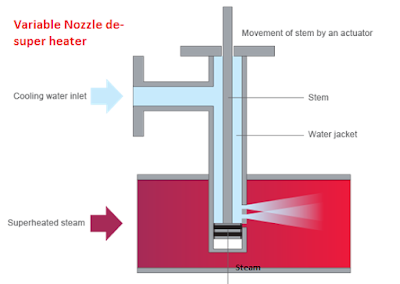### Why do the vibrations occur in machines???

What do you mean by the term VIBRATION?
A periodic motion of the particles of an elastic body or medium in alternately opposite directions from the position of equilibrium when that equilibrium has been disturbed.
Why do the VIBRATIONS occur in machines?
Vibrations in the machine due to:
• Misalignment of the driver & driven equipments
• Bearing failure or more clearance in bearing
• Unbalance in machine
• Loose parts in machine
• Loose foundation bolts
• Lack of lubricant
• Low viscosity or high temperature of lubricants
• Bent shaft or more run out of shaft
• Operation of machine in critical speed band
• Wrong design of machine or its parts
• Wrong installation of machine & accessories
What are the forces responsible for vibrations in a machine?
Three basic types of forces which cause vibrations in a machine:
• Impact – loose parts, hammering in a piping system, rolling element in a bearing hitting a spall.
• Periodic – repetitive force such as unbalance or misalignment.
• Random – varies with time, for example, turbulence in piping, pump cavitations.
Each type of force produces a different reaction in the machine.
What are the effects of VIBRATION on machine?
• Bearing failure
• Coupling failure
• Machine parts failure
• Internal rubbing & seizing related problems
• More lubricants consumption
• More power consumption
• More noise
• Machine is required to run at lower loads & hence lesser machine efficiency
• Eventually machine will fail

If a machine has higher vibration in only vertical/horizontal/axial direction then what does it indicate?
• If there is more vertical vibration and other direction vibrations are minimum, then indicates looseness in machine components.
• If there is more horizontal vibration and other direction vibrations are minimum, then indicates unbalance of rotating part.
• If there is more axial vibration and other direction vibrations are minimum, then indicates misalignment.

Methods of Vibration measurement:
In power plants, generally equipments vibrations are measured in displacement & velocity
Let us discuss on the methods of vibrations measurement
1-Displacement amplitude:
Displacement amplitude measures the distance the vibrating part travels in one direction from a reference position during oscillations. (Note that the peak-to-peak displacement value, which measures total travel in both directions, is sometimes used.) This vibration measurement is important because vibrations with high displacement amplitude can cause machine components to exceed their yield point and experience catastrophic failure. Displacement measurements are typically used when vibration frequencies are low.
2-Velocity amplitude
Velocity amplitude measures the speed of the oscillation. This measurement is typically considered the industry standard for evaluating the condition of a machine based on its vibrations; because it takes into account both vibration frequency and displacement. (Recall that velocity is the rate of change of displacement.) In fact, ISO standards refer to velocity amplitude when specifying the severity of machine vibration. Velocity amplitude can be expressed in terms of peak value or, more often, in terms of the root mean square (RMS) value, which is an indicator of the vibration energy.
3-Acceleration amplitude
Acceleration amplitude is directly related to the force imparted by the vibration and is especially useful for assessing the likelihood of fracture for equipment that rotates at high speed. The high forces associated with acceleration can also cause lubrication breakdown, which can lead to excessive wear, heat, and premature failure. Acceleration is typically measured in “g,” or multiples of earth’s gravitational acceleration.
Units of vibrations measurement:
• Displacement: mm, microns, mils
• Velocity: mm/sec., inch/sec.
• Acceleration: mm/sec2.
How do you avoid machine VIBRATIONS?
Vibrations can be avoided by
• Running the machine/equipment at or below the rated load
• Following preventive maintenance regularly
• Following Condition based maintenance (CBM) regularly
• Replacing worn-out parts timely
• Implementing proper design & installation methods
• Following lubrication schedule timely with correct quantity & quality lubricants
• Following precision alignment (Rim & Face type) method
Relation between displacement, velocity & acceleration & their conversions

Velocity = (2pi X RPM/60) X Displacement (microns peak-peak)

Acceleration = (2pi X RPM/60) X Velocity (mm/sec. pk)

Acceleration = 4 pi X (RPM/60) X Displacement (microns pk-pk)
What is the critical speed of a machine? On what factors critical speed of a machine depends?
Critical speed of a shaft is the condition, where the number of natural vibrations or natural frequency equals the shaft speed in rpm.
At this speed rotating shaft becomes dynamically unstable and vibrations occur.
Critical speed depends on,
• Shaft speed
• Distance between the supports
• Type of support

Guidelines for selection & installation of machine foundations:
• For centrifugal machines, Mass of the foundation = 3 X Mass of the machine
• For reciprocating machines, Mass of the foundation = 5 X Mass of the machine
• The top of the foundation block should be at least 12” above the finished floor level
• The width of the foundation should be 1.25 to 1.5  X Vertical distance from the base to the machine centre.
Thumb rules for steam Turbine displacement vibrations measurement:
• Normal vibrations in microns = 2400 / √Turbine speed in RPM
• Alarm vibration in microns = 4500 / √Turbine speed in RPM
•  Trip vibrations in microns = 6600 / √Turbine speed in RPM

#### 1 comment:

1.Much informative sir.

Hi all,